# Make video games in Google Sheets using only built-in functions (Part 2: Board Games)

Tuesday, June 29, 2021

Hello! 👋 Hopefully you're coming here from Part 1 of this series, but just in case, here's a super quick recap:

• Enabling Google Sheets's "Iterative Calculation" feature lets us build really complex formulas without additional scripting.
• With it, we can turn checkboxes into buttons and move numbers around dynamically.
• We can then use those numbers to map "actors" to a two-dimensional grid, allowing for simple video game creation.

Another great feature in Google Sheets is the ability to share your spreadsheets with others, and edit them simultaneously. If we've already built a single-player game in a spreadsheet, why not multiplayer? Grid-based board games like chess or checkers are natural fits for a spreadsheet format, though today we'll make a fairly broad system that you can manipulate to fit the game of your choice.

A note before diving in: My goal is to make this as beginner-friendly as I can, but this post will assume at least a passing familiarity with Google Sheets and Google Sheets formulas. Like I mention in Part 1, we're intentionally doing things the hard way here, so this is sort of like jumping into the deep end if you've never played with complex formulas before. To help keep everything straight, all of the formulas I mention below will be based on an example spreadsheet that I'll link to in a minute. If you run into any questions, I'm always happy to chat!

## Setting up the board

To get started, we'll want to set up a spreadsheet that is very similar to the example from part 1:

• Turn on Iterative Calculation
• Make the cells into squares
• Set aside sections for constants, button calculation, variables, the "backstage screen" that maps pieces onto the 2D space, and the "screen" the user will interact with

The major difference now is we're going use checkboxes to fill in the "screen" the user interacts with. We're also going to add functions in the buttons section so that we can track where the user is clicking on the board. That screen is going to look a bit weird for a while, and we'll really rely on the "backstage screen" to see where our pieces are, but we'll clean it all up with Conditional Formatting at the end.

Here's a template with an 8x8 board that you can copy to get started!

Any time I reference a cell in guide below, I'll be using that template as a reference, so you can play along!

## Picking up the pieces

In our variables section, let's write down information about all of the pieces, giving them each a:

• Unique ID: I like using the alphabet for this, so the first piece is A, second is B, and so on.
• Name: This isn't always required, but if you're making a game like chess, it's handy to remember which piece is the knight, rook, etc.
• Starting X and Y: When the game starts, where should this piece be placed?
• Current X and Y: Where is the piece currently?
• Where can this piece move?: This is where the most complex formulas are going to go, and I'll dedicate a whole section to it later on in this post. Leave it blank for now.
• Coordinates: Combine the current X and Y values into a single, comma-separated string: "x,y"
• ID: Just the piece's unique ID again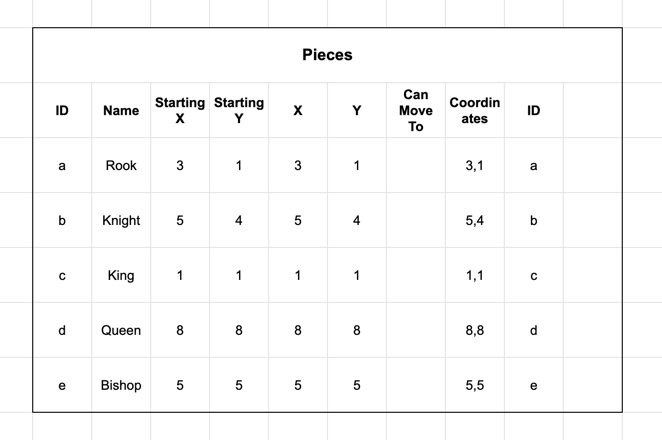While the Starting X and Y are static values that I've written in, the current X and Y will be variable. For now, let's set them to the starting values if the "reset" button (included in the template above) has been clicked:

``````=IF(reset,D27,F27)
``````

Because of our layout, you can write that in the first "X" cell, then click-and-drag to apply it to the rest: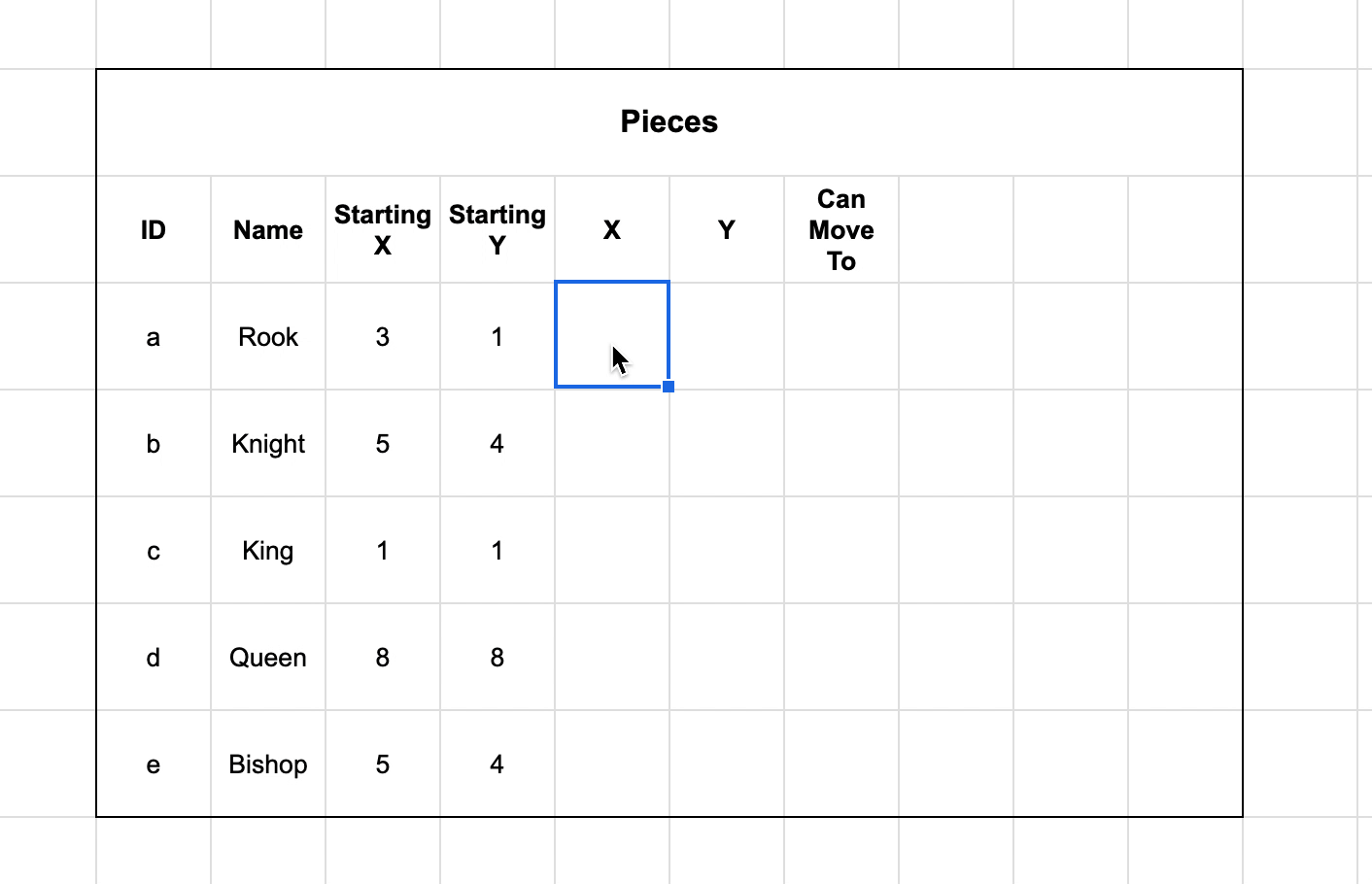Now that we have those set, we can start visualizing them on the board!

First, click the "reset" button, so that the X and Y values update to match the starting cells. Then, in the "backstage screen", let's add a VLOOKUP formula in each cell. We're specifically going to try and find a match for the Coordinates column in our pieces, then return the ID. We placed those values to the right of the X and Y columns, to make sure we're always using the latest piece positions.

I've added a named range called `pieceCoordinates` that contains the Coordinates and ID columns for our pieces, to help write this VLOOKUP:

``````=IFNA(VLOOKUP(C\$38&","&\$B39,pieceCoordinates,2,FALSE),0)
``````

The exact cell references may look slightly different based on your setup, but we're trying to match the numbers above and to the left of the "screen" with the X and Y of a piece: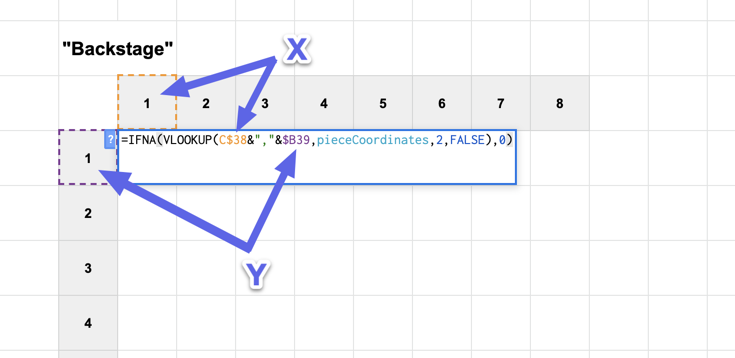Using the dollar sign also keeps the row or column static in our formula, so you can click-and-drag the single formula across the whole board while ensuring their formulas continue to reference the correct X and Y values: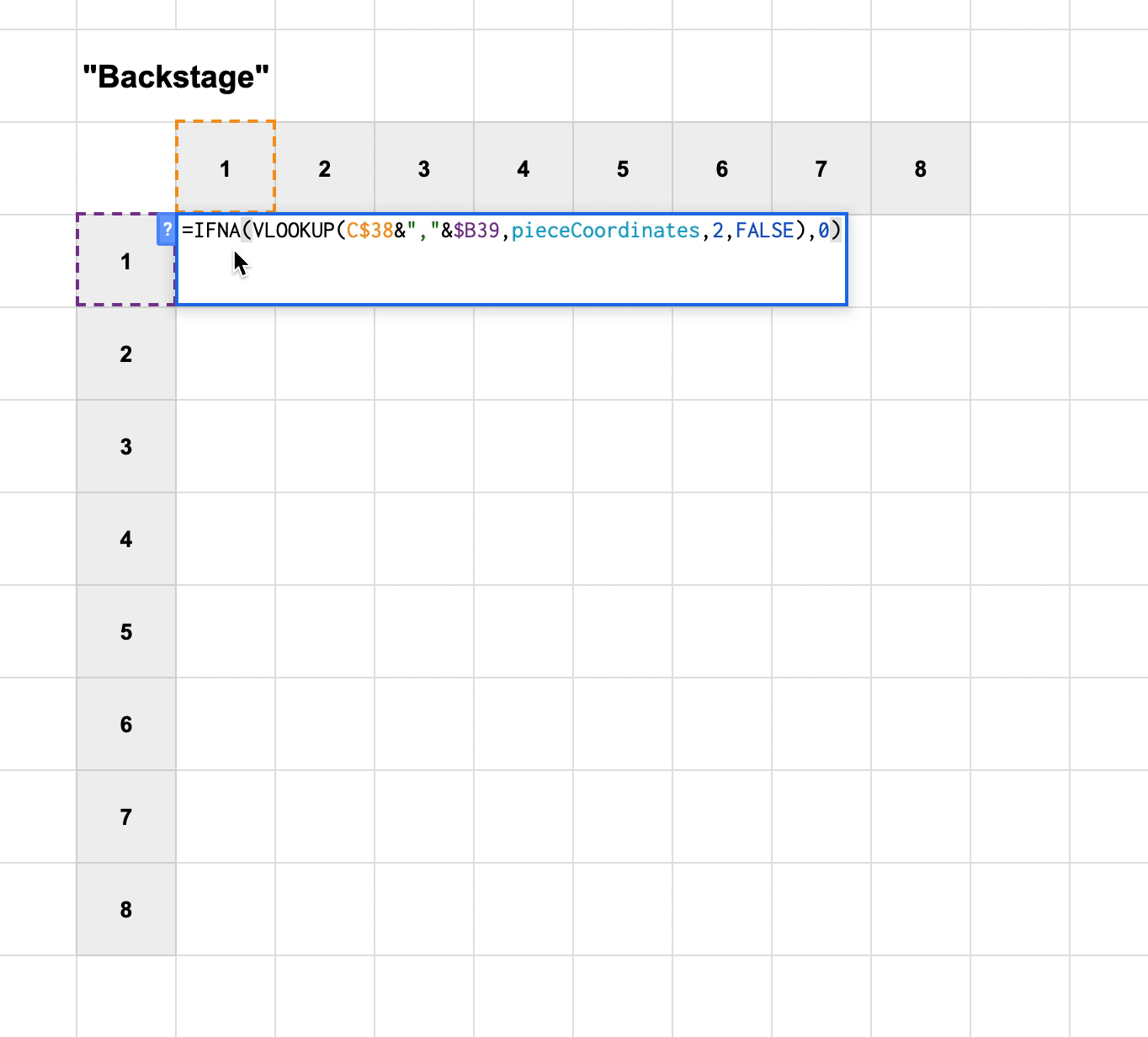Note: There are some cases where you'd want to use a QUERY formula instead of VLOOKUP, like I mentioned at the end of Part 1. The reason we're not using it here is that VLOOKUP is a "quicker" function. While QUERY has a lot of great features, it can sometimes cause your spreadsheet to slow down a little, so that not all of your spaces update in time. If you find that you don't like the VLOOKUP method, I encourage you to give QUERY a try!

## Selecting pieces

What you should have now is a board that shows where the pieces are, and a "screen" of checkboxes, with calculations that let you see which space was clicked last.

To move pieces around the board, we're first going to need to find the X and Y coordinates of that "clicked" space. There's a few ways to do this, but I've come to like this method:

In the button calculation, let's turn the middle section, which checks to see if the left side is not equal to the right (check out my guide on buttons if you're lost), and turn it into either 1s or 0s. You can do that really quickly with the N formula, which turns TRUE into 1 and FALSE into 0. If you're using this template, that formula is already added!

This means that the most recent space the user has interacted with will show a 1, while the rest will show a 0.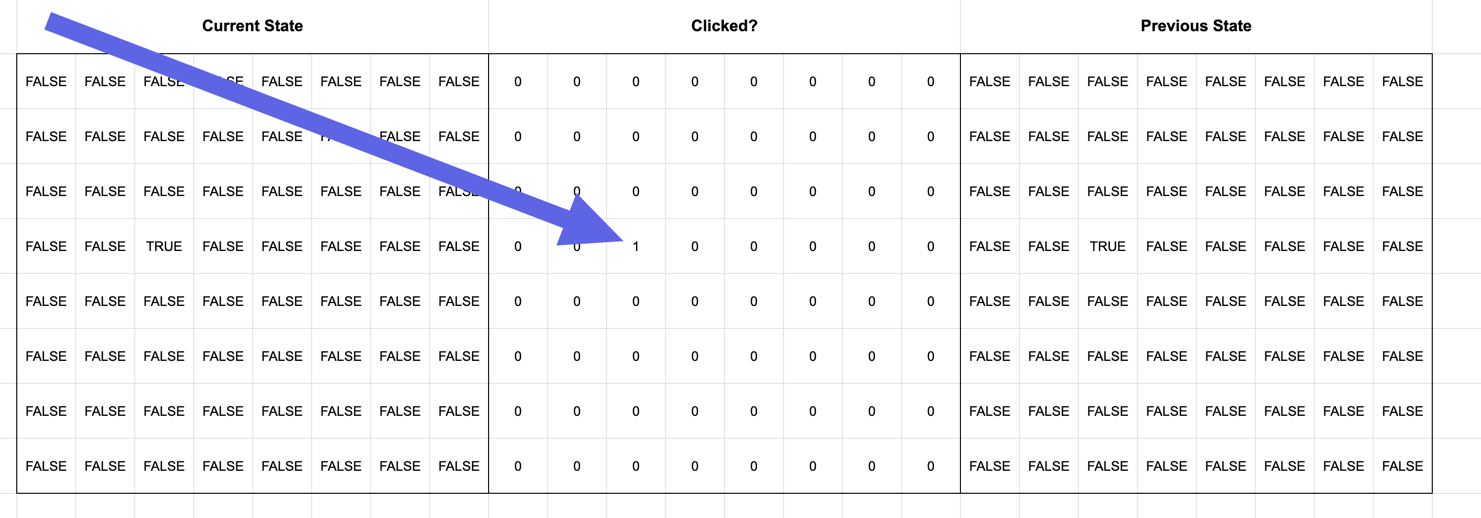And we can find the position of that space by JOINing the rows together, and FINDing the first "1". If none are found, let's return a blank instead:

``````=IFERROR(FIND("1",JOIN("",J8:Q8,J9:Q9,J10:Q10,J11:Q11,J12:Q12,J13:Q13,J14:Q14,J15:Q15)),"")
``````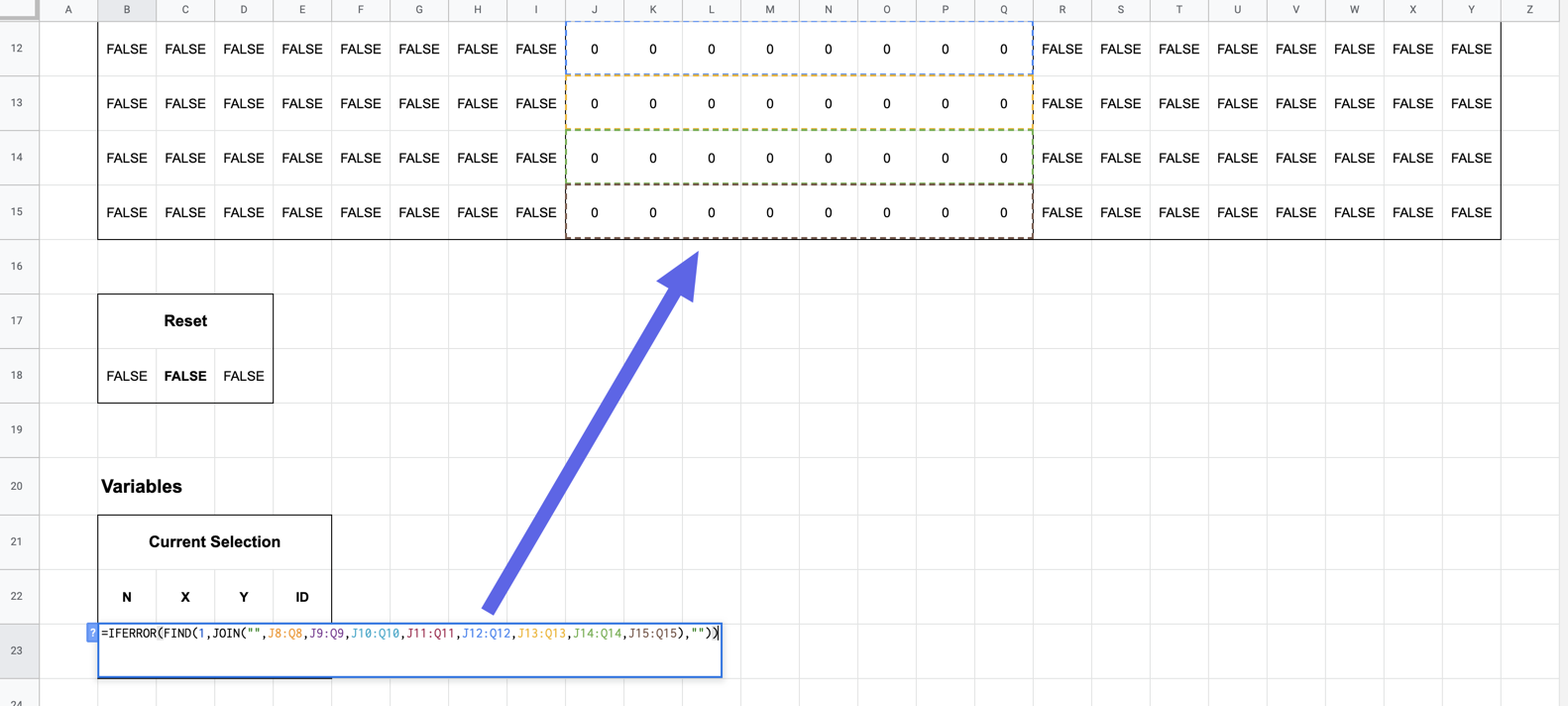"But Tyler," you may be thinking, "That only gives us a single number, not X and Y coordinates." And you would be right to think that! The number we see now is where the piece would be if we took the rows out of the board, and arranged them all in a straight line. To turn that back into our two-dimensional board, we can use some very special math.

To find the X position, we'll want to use the MOD (modulus) formula. This gives us the remainder left over when we divide two numbers. If we get the modulus of our new "selection" number and the width of our board, the result will be either the piece's X position on the board, or 0. If it's 0, that means we're on the right-hand side of the board, and should use the board's width instead.

I've gone ahead and saved a named range of the selection called `selection`, and here's what that looks like with our 8x8 example board:

``````=IF(selection="","",IF(MOD(selection,8)=0,8,MOD(selection,8)))
``````

Our calculation for Y is much simpler, as we just want to divide the selection number by the board width, and use CEILING to round up:

``````=IF(selection="","",CEILING(selection/8))
``````

Now we know exactly where the user is clicking on the board! And, because of that, we can learn which piece they're clicking on. To do that, let's add a QUERY formula that looks for any piece that matches the X and Y position of our latest selection. I've added a named range for the `pieces` to help us locate that:

``````=IF(selection="","",IFNA(QUERY(pieces,"SELECT B WHERE F="&C23&" AND G="&D23&" LIMIT 1"),""))
``````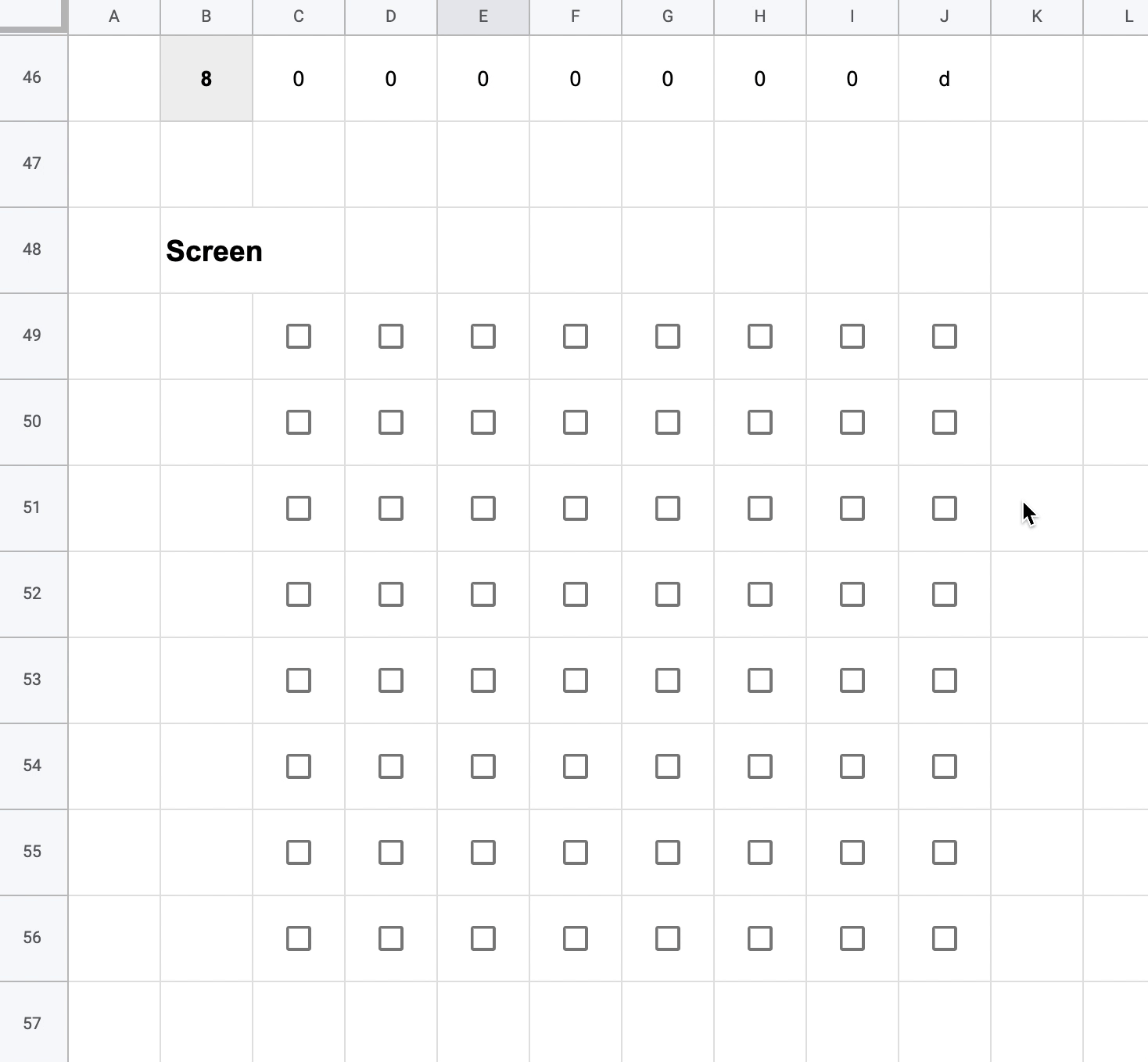Now we'll want to keep track of which piece we selected previously, so that we can see if that piece needs to move the next time the user clicks on the board.

Below your piece information, add a new space for the previously selected piece. We'll use effectively the same QUERY formula as up above, but grab the piece ID, Name, X, Y, and Can Move To columns. This way, when our pieces are figuring out whether they need to move, they can look here to see if they were the most recently-selected piece.

``````=IFNA(QUERY(pieces,"SELECT B,C,F,G,H WHERE B='"&E23&"' LIMIT 1"),{"","","","",""})
``````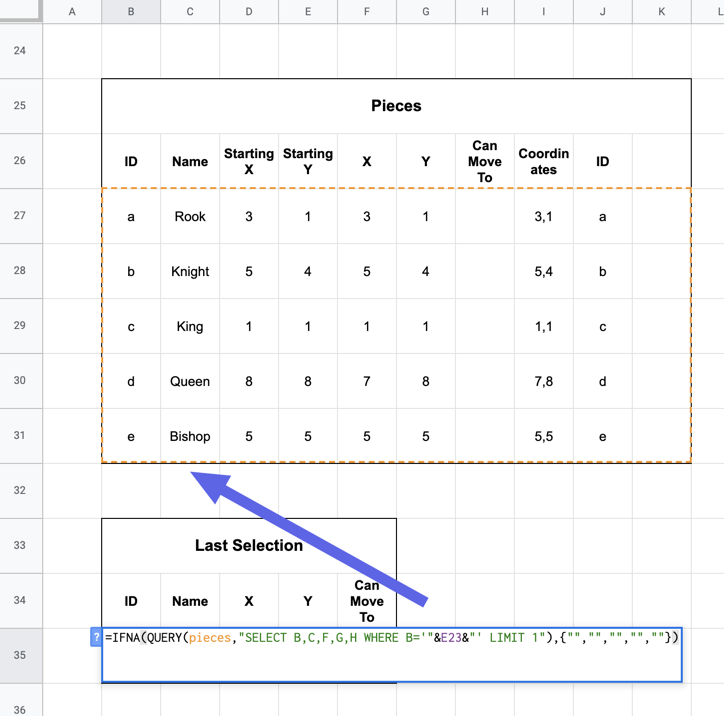Using that, we can go back through our X and Y columns in the `pieces` range, and update them to see whether that piece was selected previously, and if so, update the X and Y to match the new selection:

``````=IF(reset,D27,IF(lastSelection=\$B27,C\$23,F27))
``````

Now if we select a piece on the screen, then select another space, the piece will move!

I added a little bit of conditional formating here to help make it more obvious: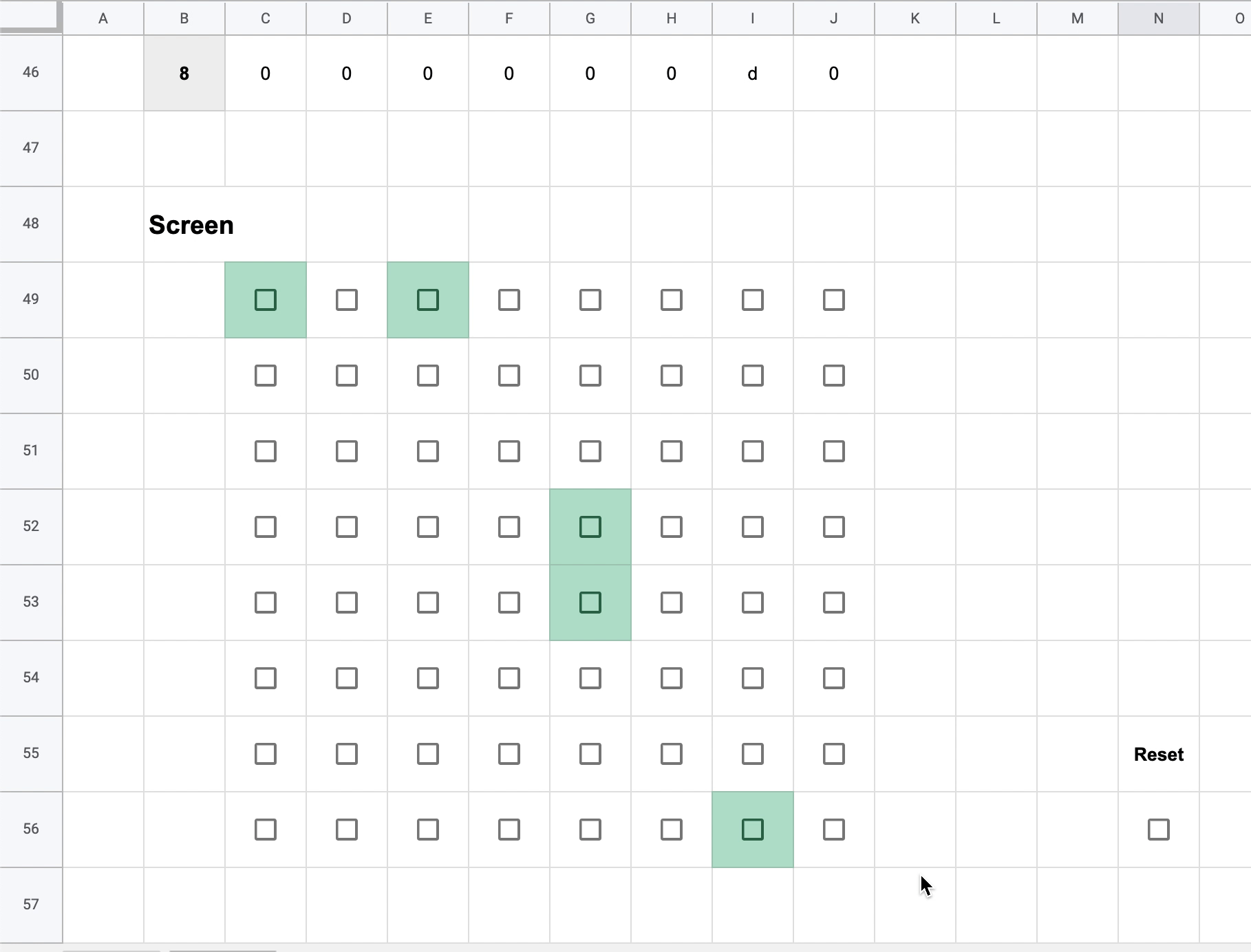## Determining where pieces can move

After completing the last step, we can move a piece anywhere on the board, including on top of other pieces. This is great if you're playing something free-form, or keeping track of your Dungeons & Dragons characters virtually, but not so great if you want to play something with, you know, rules.

In this section, we're going to tell each piece where it can move to, and restrict them to just those spaces.

This section takes a lot of patience and nesting IF statements. If you've been reading this post straight through, I'd recommend taking this opportunity to stretch and get a drink of water!

In the `pieces` range that we set aside, we've left the Can Move To column blank so far. That's because we're going to write formulas that take the current X and Y position of each piece, then determine rules that dictate where each piece can go. Based on those rules, we want a list of coordinates, listing each square the piece can move to.

Example: Let's say we have a piece that can move one space in any orthogonal direction (up, down, left, right). If it is currently at 2,2, we would want the "Can Move To" value for that piece to look something like this:

``````2,1;3,2;2,3;1,2;
``````

That list contains the X and Y values of a space the piece can move to, with each pair separated by a semicolon (or any character of your choice).

To achieve that result, we're first going to create a new named range of our "backstage" screen, including the numbers on the left, and call it `board`: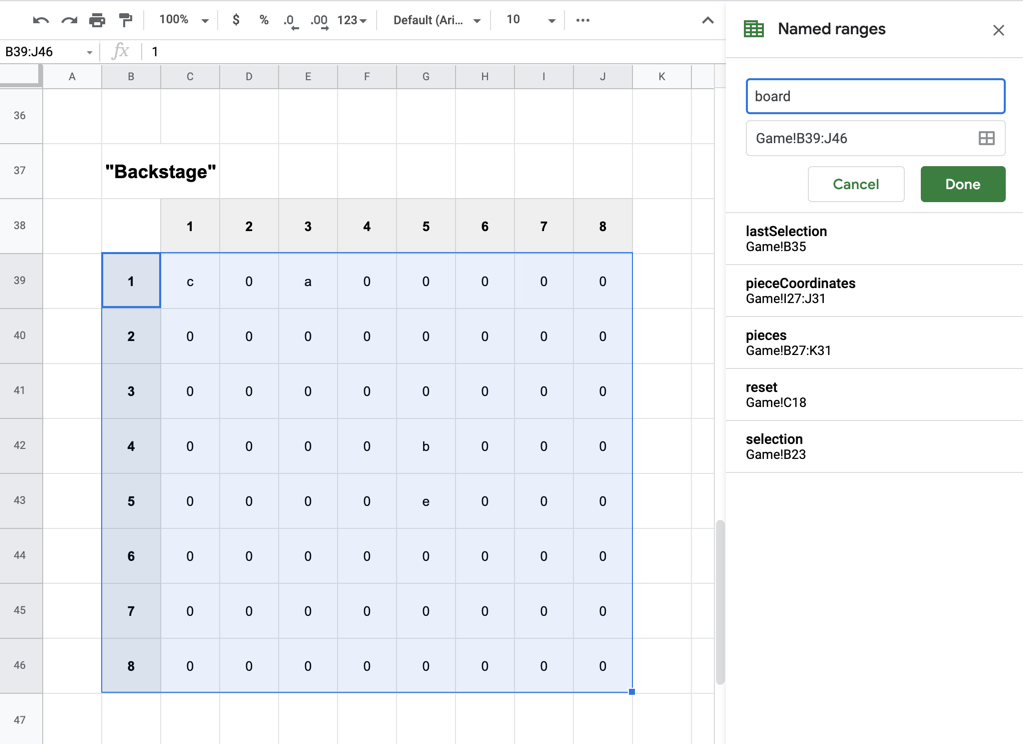That's going to let us refer to spaces on the board in the next step, where we write a series of IF formulas that check the current piece, and see if the spaces it wants to move to are empty, to build out that list.

The complexity of this can vary depending on what kind of game you're making. For example: in checkers, all of the pieces have the same rules for movement, but in chess they vary based on the type of piece. To help illustrate this, I'm going to write out the functions for two chess pieces: the knight and the rook.

### Example 1: The Knight

In chess, the knight can move two spaces in any orthogonal direction, turn 90 degrees, then move one space in that direction. It can also "jump" over other pieces, meaning it won't matter if another piece gets in the way. Puzzles like The Knight's Tour help illustrate its versatility really well.

Because the knight doesn't care about other pieces, it's one of the simpler examples for our purposes. What we're going to do is write a series of IF statements that check to see whether a destination space is empty, and return the X,Y coordinates of that space if it is. We'll then use the `&` to concatenate all of the results into a list of coordinates.

Using our example template, I have the knight's X and Y positions in cells F28 and G28, respectively. Here's the formula for checking just one destination that is two spaces up and one space to the right of the piece's current position:

``````=IF(IFERROR(VLOOKUP(G28-2,board,F28+2)=0,FALSE),F28+1&","G28-2&";","")
``````

A few things to note there:

• We're performing a VLOOKUP formula to look at the board. This is why we include the numbers on the left in our named range, as that lets us use the piece's current Y position to find the right row, then locate the piece's X position in that row.
• We add an additional 1 to the piece's X in our VLOOKUP formula, to account for the numbers on the left.
• The IFERROR formula is included in case we try to look up a space that is not on the board, which would normally return an error in VLOOKUP.
• If the space is not empty, or if VLOOKUP returns an error, we want to return a blank, or "". That way, we only list the viable spaces.

Using ampersands, we can concatenate that formula with 7 more just like it, to look at all of the spaces a knight might move to:

``````=IF(IFERROR(VLOOKUP(G28-2,board,F28+2,FALSE)=0,FALSE),F28+1&","&G28-2&";","")
&IF(IFERROR(VLOOKUP(G28-2,board,F28,FALSE)=0,FALSE),F28-1&","&G28-2&";","")
&IF(IFERROR(VLOOKUP(G28-1,board,F28+3,FALSE)=0,FALSE),F28+2&","&G28-1&";","")
&IF(IFERROR(VLOOKUP(G28-1,board,F28-1,FALSE)=0,FALSE),F28-2&","&G28-1&";","")
&IF(IFERROR(VLOOKUP(G28+1,board,F28+3,FALSE)=0,FALSE),F28+2&","&G28+1&";","")
&IF(IFERROR(VLOOKUP(G28+1,board,F28-1,FALSE)=0,FALSE),F28-2&","&G28+1&";","")
&IF(IFERROR(VLOOKUP(G28+2,board,F28+2,FALSE)=0,FALSE),F28+1&","&G28+2&";","")
&IF(IFERROR(VLOOKUP(G28+2,board,F28,FALSE)=0,FALSE),F28-1&","&G28+2&";","")
``````

To make this easier to read in Google Sheets, I like to add line returns within the formula, which you can do with `alt + enter`

When we run this formula in our sheet, the list will update itself to match the spaces that the knight can move to, no matter where it is on the board: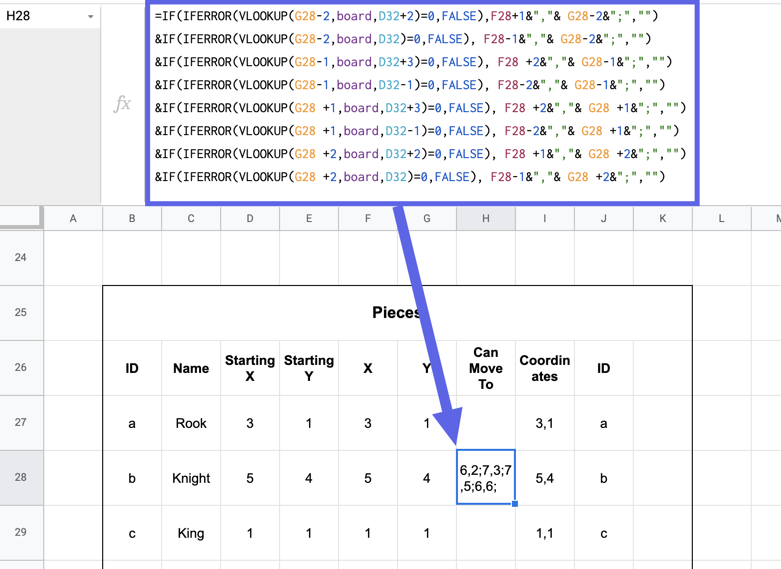### Example 2: The Rook

The rook is a slightly more straightforward piece, as it can only move up, down, left, or right. However, it can move as many spaces as it wants unless there is another piece in the way. This means that to determine where it can move, we need to look at each space in a certain direction, check if it is free, and stop if it is not. This is where we get into nested IF formulas!

Here is an example for the rook in my example board, which has its X and Y at cells F27 and G27, to check the two spaces to the right of it:

``````=IF(IFERROR(VLOOKUP(G27,board,F27+2)=0,FALSE),F27+1&","&G27&";"
&IF(IFERROR(VLOOKUP(G27,board,F27+3)=0,FALSE),F27+2&","&G27&";"
``````

Using this method, we check to see if the space to the piece's immediate right is free. If it is, we go on to check if the space to the right of that is free, and so on. We keep adding to that list as far down as we want to go, and as soon as one IF runs into a piece or the edge of the board, the formula stops.

So far so good, right? OK, buckle in. Here's what it looks like when we check eight spaces in each direction the rook can go:

``````=IF(IFERROR(VLOOKUP(G27,board,F27+2,FALSE)=0,FALSE),F27+1&","&G27&";"
&IF(IFERROR(VLOOKUP(G27,board,F27+3,FALSE)=0,FALSE),F27+2&","&G27&";"
&IF(IFERROR(VLOOKUP(G27,board,F27+4,FALSE)=0,FALSE),F27+3&","&G27&";"
&IF(IFERROR(VLOOKUP(G27,board,F27+5,FALSE)=0,FALSE),F27+4&","&G27&";"
&IF(IFERROR(VLOOKUP(G27,board,F27+6,FALSE)=0,FALSE),F27+5&","&G27&";"
&IF(IFERROR(VLOOKUP(G27,board,F27+7,FALSE)=0,FALSE),F27+6&","&G27&";"
&IF(IFERROR(VLOOKUP(G27,board,F27+8,FALSE)=0,FALSE),F27+7&","&G27&";"
&IF(IFERROR(VLOOKUP(G27,board,F27+9,FALSE)=0,FALSE),F27+8&","&G27&";"
,""),""),""),""),""),""),""),"")
&IF(IFERROR(VLOOKUP(G27,board,F27,FALSE)=0,FALSE),F27-1&","&G27&";"
&IF(IFERROR(VLOOKUP(G27,board,F27-1,FALSE)=0,FALSE),F27-2&","&G27&";"
&IF(IFERROR(VLOOKUP(G27,board,F27-2,FALSE)=0,FALSE),F27-3&","&G27&";"
&IF(IFERROR(VLOOKUP(G27,board,F27-3,FALSE)=0,FALSE),F27-4&","&G27&";"
&IF(IFERROR(VLOOKUP(G27,board,F27-4,FALSE)=0,FALSE),F27-5&","&G27&";"
&IF(IFERROR(VLOOKUP(G27,board,F27-5,FALSE)=0,FALSE),F27-6&","&G27&";"
&IF(IFERROR(VLOOKUP(G27,board,F27-6,FALSE)=0,FALSE),F27-7&","&G27&";"
&IF(IFERROR(VLOOKUP(G27,board,F27-7,FALSE)=0,FALSE),F27-8&","&G27&";"
,""),""),""),""),""),""),""),"")
&IF(IFERROR(VLOOKUP(G27-1,board,F27+1,FALSE)=0,FALSE),F27&","&G27-1&";"
&IF(IFERROR(VLOOKUP(G27-2,board,F27+1,FALSE)=0,FALSE),F27&","&G27-2&";"
&IF(IFERROR(VLOOKUP(G27-3,board,F27+1,FALSE)=0,FALSE),F27&","&G27-3&";"
&IF(IFERROR(VLOOKUP(G27-4,board,F27+1,FALSE)=0,FALSE),F27&","&G27-4&";"
&IF(IFERROR(VLOOKUP(G27-5,board,F27+1,FALSE)=0,FALSE),F27&","&G27-5&";"
&IF(IFERROR(VLOOKUP(G27-6,board,F27+1,FALSE)=0,FALSE),F27&","&G27-6&";"
&IF(IFERROR(VLOOKUP(G27-7,board,F27+1,FALSE)=0,FALSE),F27&","&G27-7&";"
&IF(IFERROR(VLOOKUP(G27-8,board,F27+1,FALSE)=0,FALSE),F27&","&G27-8&";"
,""),""),""),""),""),""),""),"")
&IF(IFERROR(VLOOKUP(G27+1,board,F27+1,FALSE)=0,FALSE),F27&","&G27+1&";"
&IF(IFERROR(VLOOKUP(G27+2,board,F27+1,FALSE)=0,FALSE),F27&","&G27+2&";"
&IF(IFERROR(VLOOKUP(G27+3,board,F27+1,FALSE)=0,FALSE),F27&","&G27+3&";"
&IF(IFERROR(VLOOKUP(G27+4,board,F27+1,FALSE)=0,FALSE),F27&","&G27+4&";"
&IF(IFERROR(VLOOKUP(G27+5,board,F27+1,FALSE)=0,FALSE),F27&","&G27+5&";"
&IF(IFERROR(VLOOKUP(G27+6,board,F27+1,FALSE)=0,FALSE),F27&","&G27+6&";"
&IF(IFERROR(VLOOKUP(G27+7,board,F27+1,FALSE)=0,FALSE),F27&","&G27+7&";"
&IF(IFERROR(VLOOKUP(G27+8,board,F27+1,FALSE)=0,FALSE),F27&","&G27+8&";"
,""),""),""),""),""),""),""),"")
``````

Whew.

All things considered, that actually isn't that bad. In Spreadsheet Tactics, my turn-based tactics game, I wanted to account for walls and moving around corners, so each piece has 125 nested IF formulas. Here's a Twitter thread with a rough illustration.

### Restricting movement

Now that we know where our pieces can move to, we want to update the formulas in our X and Y columns, so that they update only if:

• The piece is selected

AND

• The space we clicked on is a space that the piece can move to

We already have the first part taken care of from earlier. Here's what that formula looks like right now for the rook:

``````=IF(reset,D27,IF(lastSelection=\$B27,C\$23,F27))
``````

To only let the rook move if they "Can Move To" the space, let's add an AND and FIND formula:

``````=IF(reset,D27,IF(AND(lastSelection=\$B27,IFERROR(FIND(\$C\$23&","&\$D\$23,\$H27)>-1,FALSE)),C\$23,F27))
``````

That way, if I try to move the rook diagonally… well, I can't.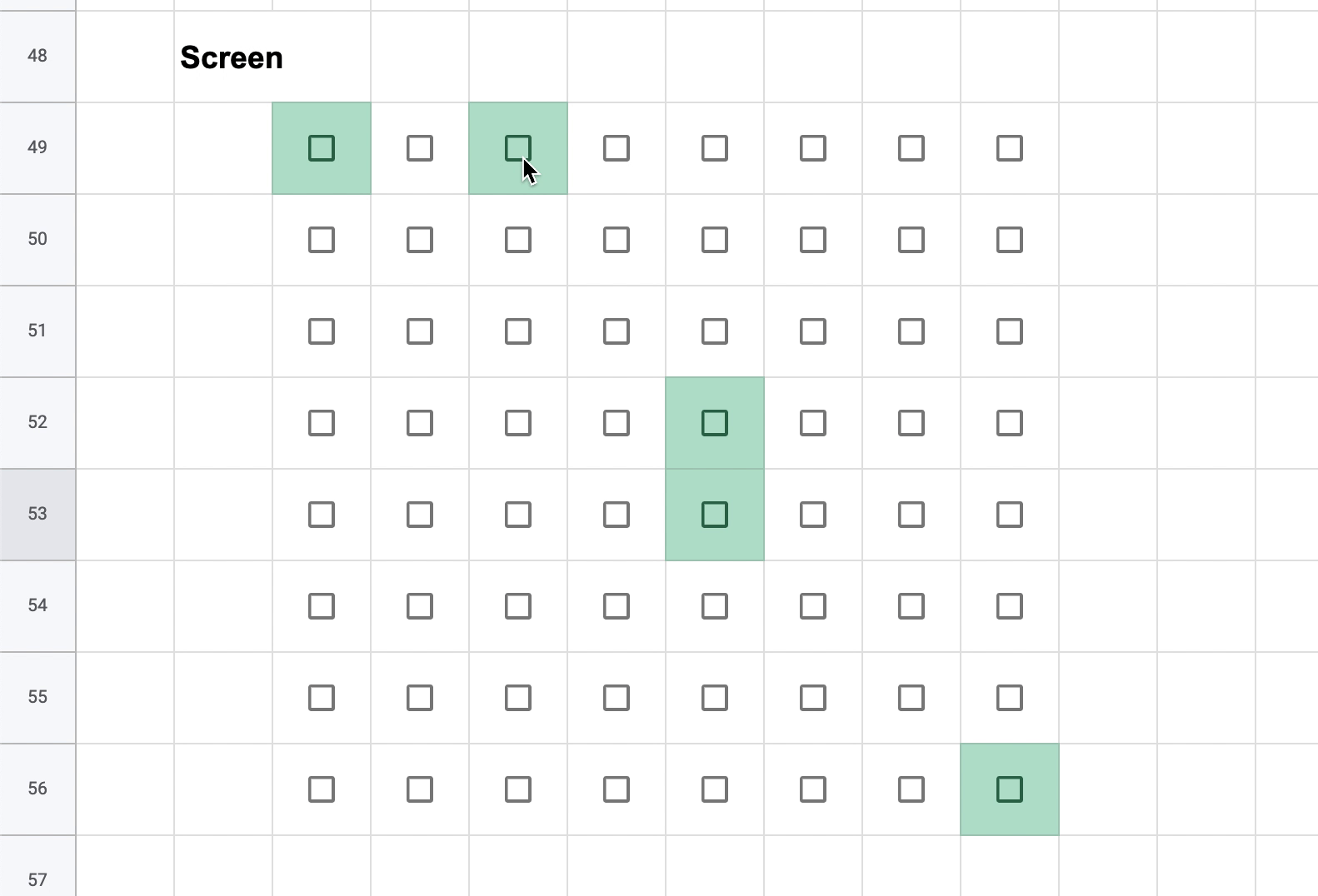Spend some time writing rules for where all of your pieces can go, and you've got 90% of a real working board game!

## Making it look good

The final 10% here is the aesthetics, and, I know. It's a spreadsheet. There's only so much we can do.

But! We're going to do our best.

The name of the game here is Conditional Formatting, and we're going to use it to color in the pieces and the spaces they can move it to. You may have done this already to point out which spaces have pieces, using a formula that checks whether the corresponding "backstage" space is not 0:

``````=C39<>0
``````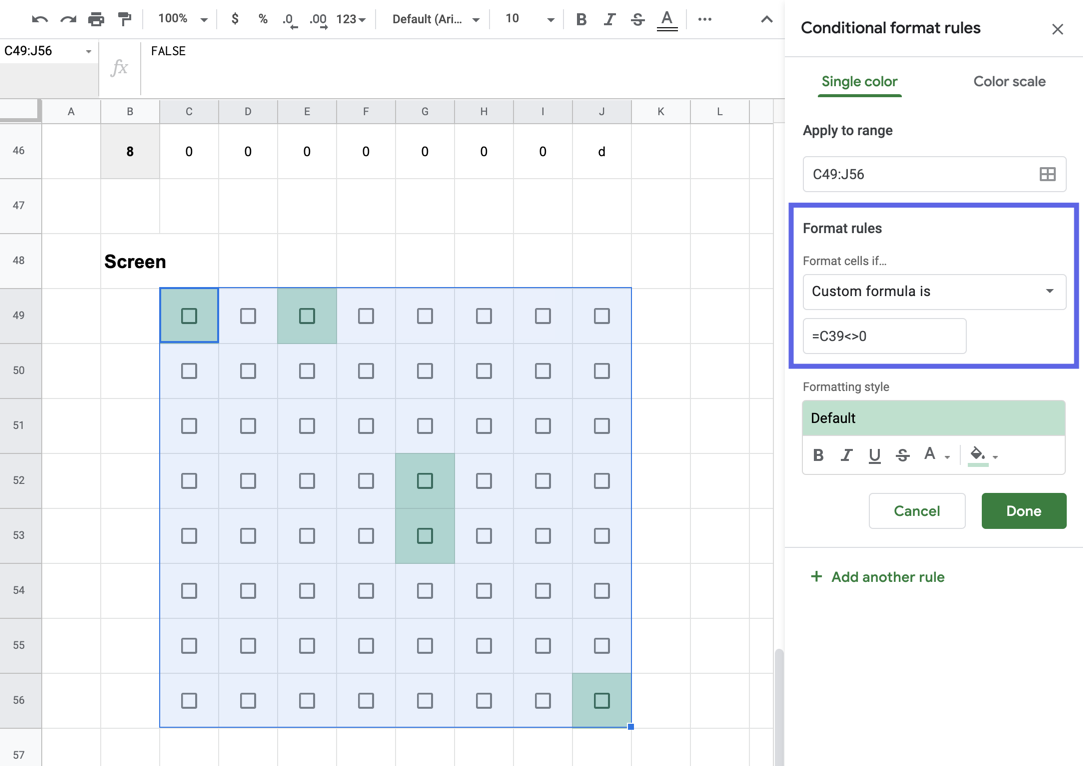We can hide the checkboxes by setting their font size to 100, and setting the colors to slight variations of each other. As an example, I'm using the custom option to set the background to `#3c78d8` and the font color to `#3d79d9`: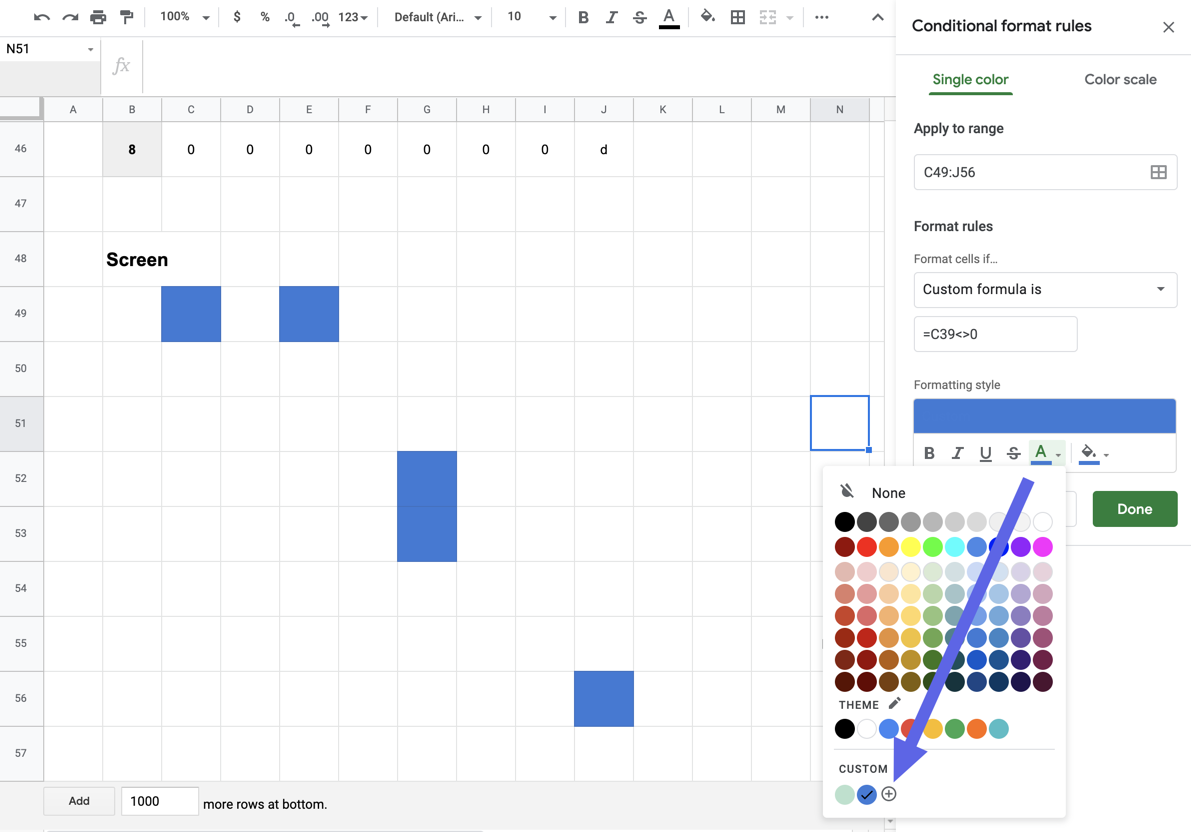To highlight spaces the piece can move to, we'll revisit the FIND formula that restricts each piece's movement, and compare the X and Y of the corresponding "backstage" piece against the current selection's "Can Move To" list:

``````=FIND(C\$38&","&\$B39,\$F\$35)>-1
``````

We can use a lighter color to point those out: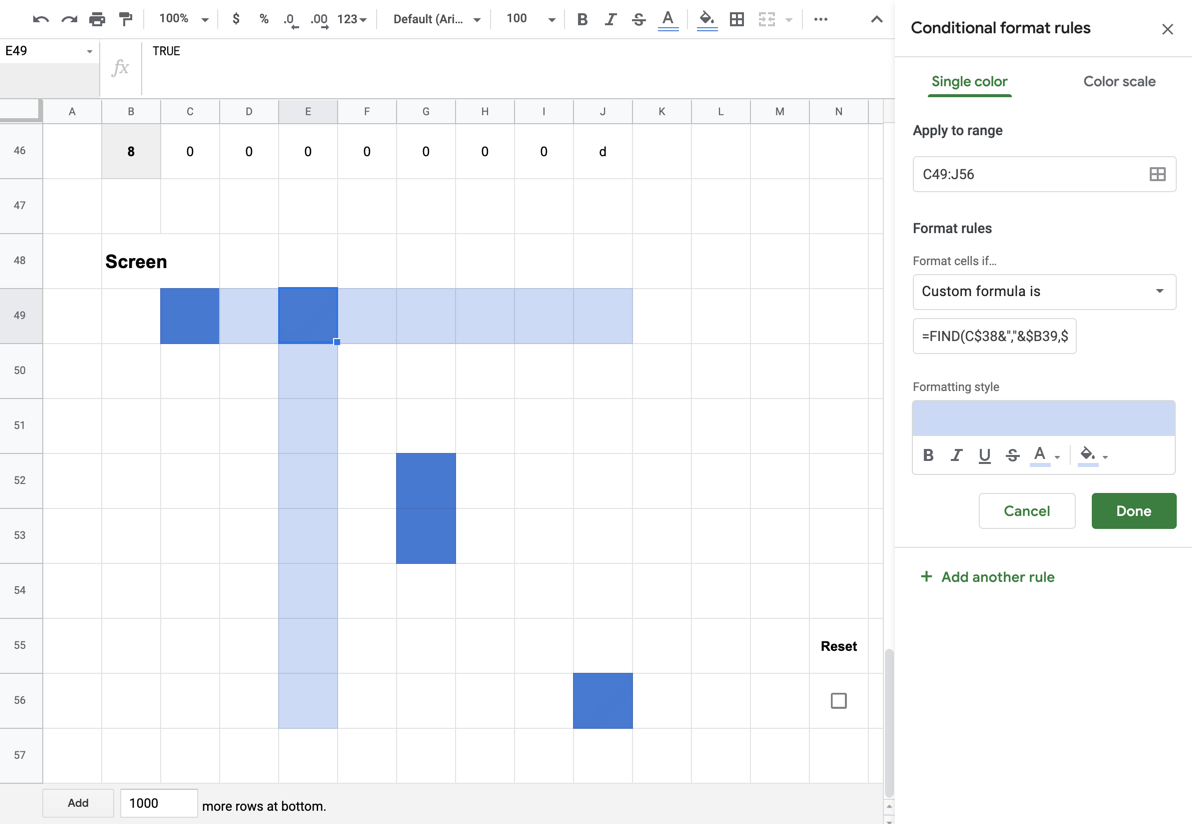Now when we click on a piece, we can see where it can move to, and move it there!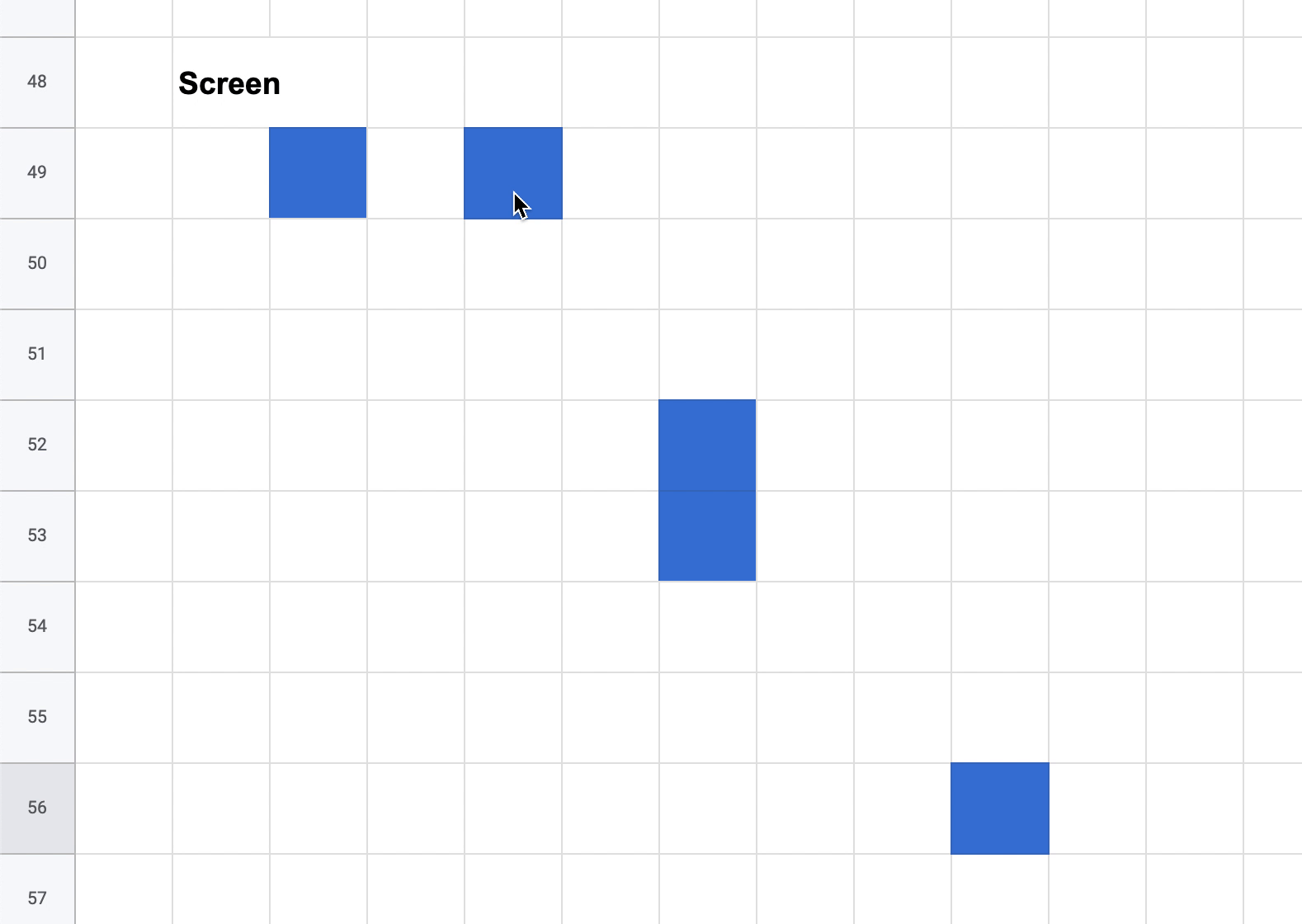To hide the spaces you've clicked on previously, set the whole board to `#fefefe` and `#ffffff`: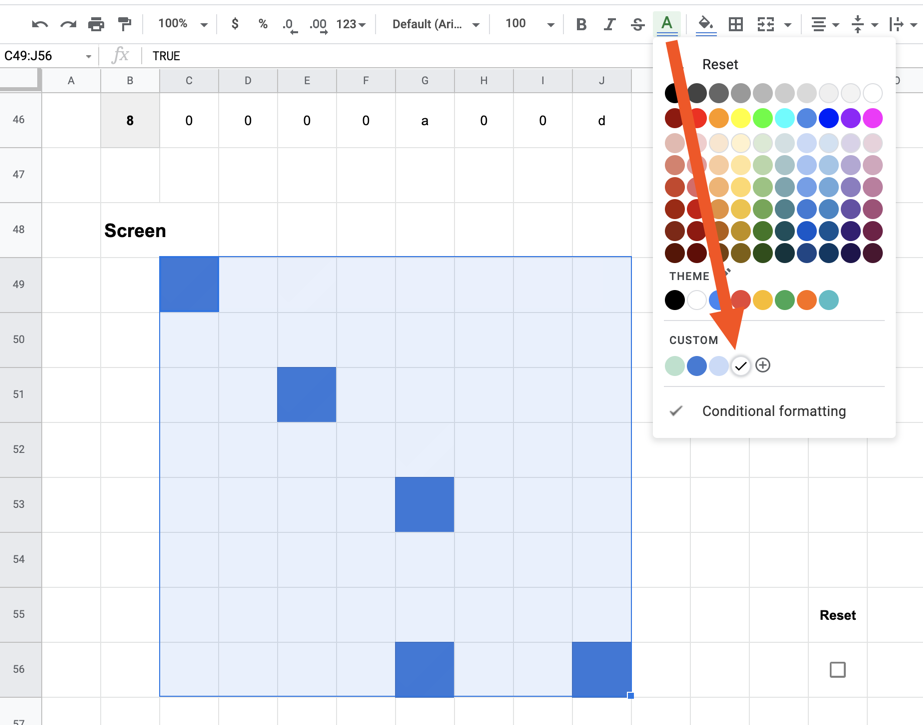Add a border around the board and hide any rows that you don't want the player to see, and you're ready to go! Here's a copy of my example, with the rook and knight ready to go.

## Taking it further

Now that you've got pieces moving around the board successfully, here are some ideas you can explore on your way towards building a full game:

• Assign pieces to different teams, and give each team a different color
• Let pieces "capture" other pieces
• Let pieces move, then perform a different action
• Make some pieces move on their own

Here are some things I've made using those ideas!

Questions, comments, gripes, complaints? Let me know!